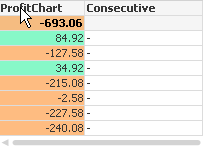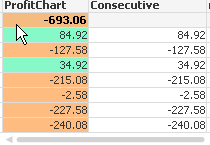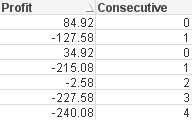Announcements
You can succeed best and quickest by helping others to succeed. Join the conversation.
cancel
Showing results for
Did you mean:Creator

## Calculating consecutive losses using above() function in a Chart

I am trying to use the above function, but I do not get any values using this

above(sum(Profit),1,3) for the Column: Consecutive. I dont understand why?It only works when I use above(sum(Profit),0,3)I do not understand why the above() function behaves like this?

What I would like to achieve is calculating how many consecutive losses I get, so this is what I would like to see:Master III

Hi Daniel,

You can use an Expression like this

If(Sum(Profit) > 0,0,Above(Column(1))+1)Regards,

AntonioCommunity Browser